# High School Math : Quadrilaterals

## Example Questions

### Example Question #5 : How To Find The Perimeter Of A Rectangle

Kayla took 25 minutes to walk around a rectangular city block. If the block's width is 1/4 the size of the length, how long would it take to walk along one length?

7 minutes

10 minutes

2.5 minutes

8 minutes

10 minutes

Explanation:

Leaving the width to be x, the length is 4x. The total perimeter is 4x + 4x + x + = 10x.

We divide 25 by 10 to get 2.5, the time required to walk the width.  Therefore the time required to walk the length is (4)(2.5) = 10.

### Example Question #9 : How To Find The Perimeter Of A Rectangle

Robert is designing a rectangular garden. He wants the area of the garden to be 9 square meters. If the length of the lot is going to be three meters less than twice the width, what will the perimeter of the lot be in meters?

10

12

6

1.5

3

12

Explanation:

Let l be the length of the garden and w be the width.

By the specifications of the problem, l = 2w-3.

Plug this in for length in the area formula:

A = l  x w = (2w - 3) x w = 9

Solve for the width:

2w²- 3w - 9 =0

(2w + 3)(w - 3) = 0

w is either 3 or -3/2, but we can't have a negative width, so w = 3.

If w = 3, then length = 2(3) - 3 = 3.

Now plug the width and length into the formula for perimeter:

P = 2 l + 2w = 2(3) + 2(3) = 12

### Example Question #6 : How To Find The Perimeter Of A Rectangle

A rectangular garden has an area of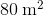. Its length ismeters longer than its width. How much fencing is needed to enclose the garden?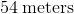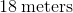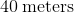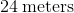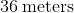Explanation:

We define the variables as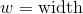and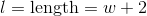.

We substitute these values into the equation for the area of a rectangle and get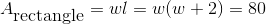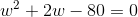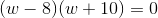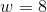or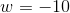Lengths cannot be negative, so the only correct answer is. If, then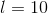Therefore,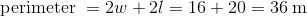.

### Example Question #1 : How To Find The Length Of The Side Of A Rectangle

The two rectangles shown below are similar. What is the length of EF?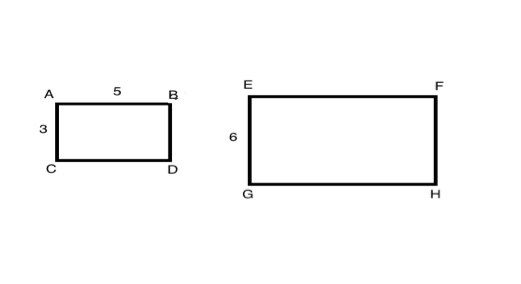5

8

10

6

10

Explanation:

When two polygons are similar, the lengths of their corresponding sides are proportional to each other.  In this diagram, AC and EG are corresponding sides and AB and EF are corresponding sides.

To solve this question, you can therefore write a proportion:

AC/EG = AB/EF ≥ 3/6 = 5/EF

From this proportion, we know that side EF is equal to 10.

### Example Question #2 : How To Find The Length Of The Side Of A Rectangle

A rectangle is x inches long and 3x inches wide.  If the area of the rectangle is 108, what is the value of x?

8

12

6

3

4

6

Explanation:

Solve for x

Area of a rectangle A = lw = x(3x) = 3x2 = 108

x2 = 36

x = 6

### Example Question #3 : How To Find The Length Of The Side Of A Rectangle

If the area of rectangle is 52 meters squared and the perimeter of the same rectangle is 34 meters. What is the length of the larger side of the rectangle if the sides are integers?

14 meters

16 meters

15 meters

12 meters

13 meters

13 meters

Explanation:

Area of a rectangle is = lw

Perimeter = 2(l+w)

We are given 34 = 2(l+w) or 17 = (l+w)

possible combinations of l + w

are 1+16, 2+15, 3+14, 4+13... ect

We are also given the area of the rectangle is 52 meters squared.

Do any of the above combinations when multiplied together= 52 meters squared? yes 4x13 = 52

Therefore the longest side of the rectangle is 13 meters

### Example Question #1 : How To Find The Length Of The Side Of A Rectangle

The width, in cm, of a rectangular fence is 2 more than half its length, in cm. Which of the following gives the width, w cm, in terms of length, l cm, of the rectangular fence?

w = 2l – 2

w = ½ l + 2

w = 2l + 2

w = ½ l – 2

w = ½ l + 2

Explanation:

To find the width, we must take half of the length, which means we must divide the length by 2. Then we must take 2 more than that number, which means we must add 2 to the number. Combining these, we get:

w = ½ l + 2

### Example Question #7 : How To Find The Length Of The Side Of A Rectangle

The width of a rectangle is 2 inches longer than 3 times its length.  Which of the following equations gives the width, w, of the rectangle in terms of its length, l,?

w = 1/3l +2

w = 6l +2

w = 3l + 2

w = 3l – 2

w = 3l + 2

Explanation:

The width equals 3 times the length, so 3l, plus an additional two inches, so + 2, = 3l + 2

### Example Question #1 : How To Find The Length Of The Side Of A Rectangle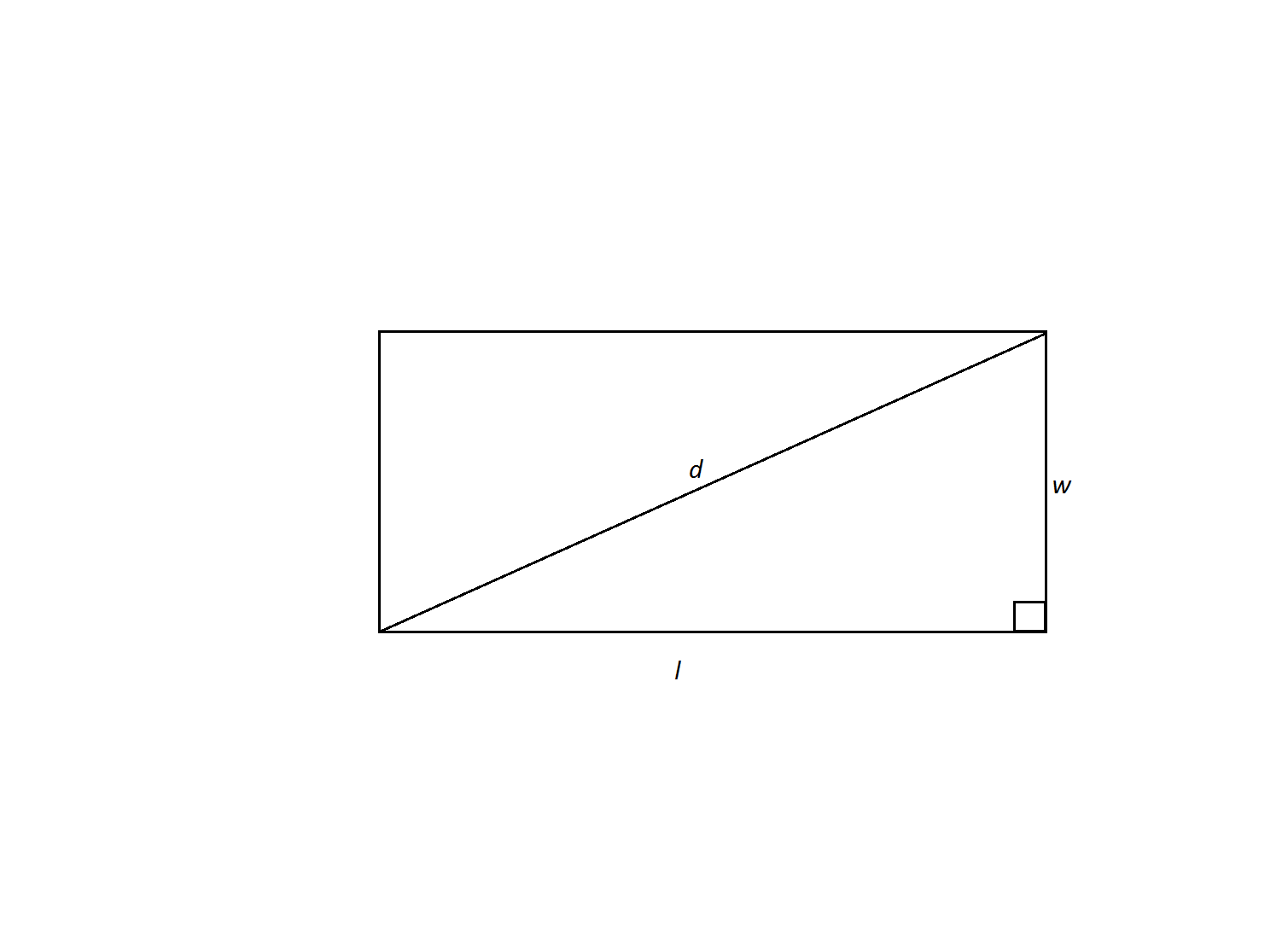A rectangle has a width of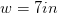and a diagonal of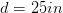. What is its perimeter?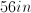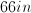Not enough information to solve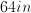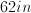Explanation:

We are given the rectangle's diagonal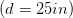and width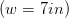and are asked to find its perimeter. The diagonal forms two right triangles within the rectangle; therefore, we can use the Pythagorean theorem to find the length of the rectangle's missing side. Then we can use the formula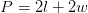to find the perimeter of the rectangle.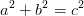In our case, we can rename the variables to match our triangle.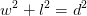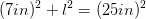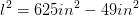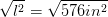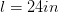Now we can calculate the perimeter.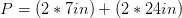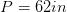### Example Question #8 : How To Find The Length Of The Side Of A Rectangle

Your dad shows you a rectangular scale drawing of your house. The drawing is 6 inches by 8 inches. You're trying to figure out the actual length of the shorter side of the house. If you know the actual length of the longer side is 64 feet, what is the actual length of the shorter side of the house (in feet)?

60

36

48

81

32